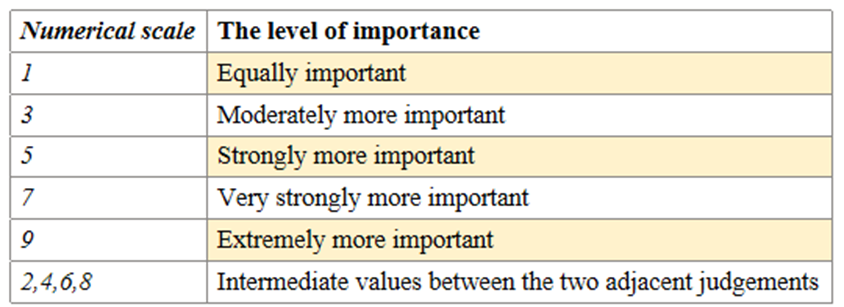top of page
• bruce yu

# Analytic Hierachy Process

Updated: Jul 31

Analytic hierarchy process, also known as AHP, helps people make decision based on multiple criteria, by the 3 sections– goal, criteria, and alternatives in a hierarchy structure. AHP quantifies the criteria and alternative options in a decision-making process and relates these elements to the goal. It works based mainly on linear algebra and calculation of judgement matrix.

In AHP, stakeholders first compare the importance of criteria, two at once, through pair-wise comparisons, to determine the weight of the criteria. Then numerical priorities are calculated for each of the alternative options. The final decision is made by calculating the total score of each option and rank them to find out the best choice.

The figure given below shows the basic steps in AHP.STEP 1: Establish Hierarchy

Level 1 Overall objective: Solar energy storage system

Level 2 Criteria: Cost, weight, volume, round-trip efficiency, battery life, and safety rating

Level 3 Options: Combinations of batteriesAn expample of Three levels in AHP

STEP 2: Establish judgement matrix

Constructing this judgement matrix involves:

1) Pair-wise comparisons of the relative importance of factors, instead of comparing all the factors at once. This method first was firstly introduced by T.L. Saaty.

2) Defining a scale of 1-9 with each scale clearly indicating a level of relative importance between the 2 factors. This increases the overall accuracy of the model.STEP 3: Single hierarchy arrangement and consistency check

An incompatible matrix means it has an incomplete consistency, which makes its credibility unacceptable for further arrangement. Hence, we need to run a consistency check.

It is hold commonly that if the consistency ratio, CR < 0.1, the judgement matrix is within an acceptable inconsistency range. After running the test, we got the value of CR, which is 0.0593, is considered within the acceptable range. This means the matrix passed the consistency test.

bottom of page